## How to Calculate and Solve for Glide Shear | Fracture Mechanics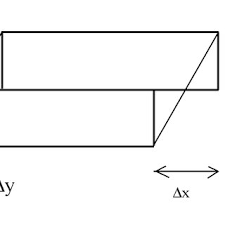The image above represents glide shear.

To compute for glide shear, two essential parameters are needed and these parameters are Stacking Fault Energy (γ) and Varying Grain Constant (M’).

The formula for calculating glide shear:

E = γ / M’

Where:

E = Glide Shear
γ = Stacking Fault Energy
M’ = Varying Grain Constant

Let’s solve an example;
Find the glide shear when the stacking fault energy is 18 and the varying grain constant is 2.

This implies that;

γ = Stacking Fault Energy = 18
M’ = Varying Grain Constant = 2

E = γ / M’
E = 18 / 2
E = 9

Therefore, the glide shear is 9 Pa.

Calculating the Stacking Fault Energy when the Glide Shear and the Varying Grain Constant is Given.

γ = E x M’

Where;

γ = Stacking Fault Energy
E = Glide Shear
M’ = Varying Grain Constant

Let’s solve an example;
Find the stacking fault energy when the glide shear is 14 and the varying grain constant is 8.

This implies that;

E = Glide Shear = 14
M’ = Varying Grain Constant = 8

γ = E x M’
γ = 14 x 8
γ = 112

Therefore, the stacking fault energy is 112.

## How to Calculate and Solve for Elongation Potential of Twin Deformation | Fracture Mechanics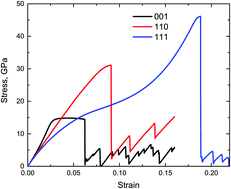The image above represents elongation potential of twin deformation.

To compute for elongation potential of twin deformation, two essential parameters are needed and these parameters are Distance between the 2 Slip Planes (S) and Angle of Deformation (x).

The formula for calculating elongation potential of twin deformation:

l’/l = (1 + Stanx)0.5

Where:

l’/l = Elongation Potential of Twin Deformation
S = Distance between the 2 Slip Planes
x = Angle of Deformation

Let’s solve an example;
Find the elongation potential of twin deformation when the distance between the 2 slip planes is 24 and the angle of deformation is 12°.

This implies that;

S = Distance between the 2 Slip Planes = 24
x = Angle of Deformation = 12°

l’/l = (1 + Stanx)0.5
l’/l = (1 + 24tan(12°))0.5
l’/l = (1 + 24(0.213))0.5
l’/l = (1 + 5.101)0.5
l’/l = (6.101)0.5
l’/l = 2.470

Therefore, the elongation potential of twin deformation is 2.470.

## How to Calculate and Solve for Partial Dislocation Separation | Fracture Mechanics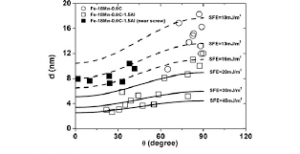The image above represents partial dislocation separation.

To compute for partial dislocation separation, four essential parameters are needed and these parameters are Shear Modulus (G), Burger Vector (b2), Burger Vector (b3and Stacking Fault Energy (γ).

The formula for calculating partial dislocation separation:

d = Gb2b3 / 2πγ

Where:

d = Partial Dislocation Separation
G = Shear Modulus
b2 and b3 = Burger Vectors
γ = Stacking Fault Energy

Let’s solve an example;
Find the partial dislocation separation when the shear modulus is 4. the burger vectors is 8 and 10, the stacking fault energy is 14.

This implies that;

G = Shear Modulus = 4
b2 and b3 = Burger Vectors = 8 and 10
γ = Stacking Fault Energy = 14

d = Gb2b3 / 2πγ
d = (4)(8)(10) / 2π(14)
d = 320 / 87.96
d = 3.64

Therefore, the partial dislocation separation is 3.64 m.

## How to Calculate and Solve for Modulus of Rupture | Fracture Mechanics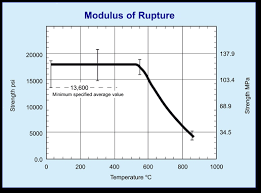The image above represents modulus of rupture.

To compute for modulus of rupture, three essential parameters are needed and these parameters are Bending Moment (M), Distance (c) and Moment of Inertia (I).

The formula for calculating modulus of rapture:

σ = Mc / I

Where:

σ = Modulus of Rupture
M = Bending Moment
c = Distance
I = Moment of Inertia

Let’s solve an example;
Find the modulus of rupture when the bending moment is 22, the distance is 10 and the moment of inertia is 14.

This implies that;

M = Bending Moment = 22
c = Distance = 10
I = Moment of Inertia = 14

σ = Mc / I
σ = 22(10) / 14
σ = 220 / 14
σ = 15.71

Therefore, the modulus of rupture is 15.71 Pa.

Calculating the Bending Moment when the Modulus of Rupture, the Distance and the Moment of Inertia is Given.

M = σ x I / c

Where;

M = Bending Moment
σ = Modulus of Rupture
c = Distance
I = Moment of Inertia

Let’s solve an example;
Find the bending moment when the modulus of rupture is 22, the distance is 8 and the moment of inertia is 2.

This implies that;

σ = Modulus of Rupture = 22
c = Distance = 8
I = Moment of Inertia = 2

M = σ x I / c
M = 22 x 2 / 8
M = 44 / 8
M = 5.5

Therefore, the bending moment is 5.5.

## How to Calculate and Solve for Shear Modulus of Rubber | Fracture Mechanics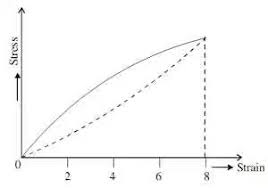The image above represents shear modulus of rubber.

To compute for shear modulus of rubber, three essential parameters are needed and these parameters are Density of Network Cross Links (N), Boltzmann’s Constant (KBand Temperature (T).

The formula for calculating shear modulus of rubber:

G = NKBT

Where:

G = Shear Modulus of Rubber
N = Density of Network Cross Links
KB = Boltzmann’s Constant
T = Temperature

Let’s solve an example;
Find the shear modulus of rubber when the density of network cross links is 21, the boltzmann’s constant is 1.38064852E-23 and the temperature is 10.

This implies that;

N = Density of Network Cross Links = 21
KB = Boltzmann’s Constant = 1.38064852E-23
T = Temperature = 10

G = NKBT
G = (21)(1.38064852e-23)(10)
G = 2.89

Therefore, the shear modulus of rubber is 2.89e-23 Pa.

Calculating the Density of Network Cross Links when the Shear Modulus of Rubber, Boltzmann’s Constant and the Temperature is Given.

N = G / KBT

Where;

N = Density of Network Cross Links
G = Shear Modulus of Rubber
KB = Boltzmann’s Constant
T = Temperature

Let’s solve an example;
Find the density of network cross links when the shear modulus of rubber is 14, the boltzmann’s constant is 1.380e-23 and temperature is 4.

This implies that;

G = Shear Modulus of Rubber = 14
KB = Boltzmann’s Constant = 1.380e-23
T = Temperature = 4

N = G / KBT
N = 14 / 1.380e-23 x 4
N = 14 / 5.52e-23
N = 2.54e-23

Therefore, the network cross links is 2.54e-23.

## How to Calculate and Solve for Resiliency | Fracture Mechanics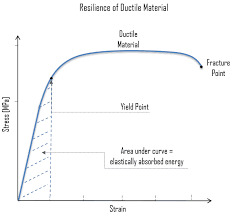The image above represents resiliency.

To compute for resiliency, two essential parameters are needed and these parameters are Maximum Stress (σmax) and Young’s Modulus of Elasticity (E).

The formula for calculating resiliency:

R = σmax² / 2E

Where:

R = Resiliency
σmax = Maximum Stress
E = Young’s Modulus of Elasticity

Let’s solve an example;
Find the resiliency when the maximum stress is 32 and the young’s modulud of elasticity is 12.

This implies that;

σmax = Maximum Stress = 32
E = Young’s Modulus of Elasticity = 12

R = σmax² / 2E
R = 32² / 2(12)
R = 1024 / 24
R = 42.67

Therefore, the resiliency is 42.67 Pa.

Calculating the Maximum Stress when the Resiliency and the Young’s Modulus of Elasticity is Given.

σmax = R x 2E

Where;

σmax = Maximum Stress
R = Resiliency
E = Young’s Modulus of Elasticity

Let’s solve an example;
Find the maximum stress when the resiliency is 15 and the young’s modulus of elasticity is 3.

This implies that;

R = Resiliency = 15
E = Young’s Modulus of Elasticity = 3

σmax = R x 2E
σmax = 15 x 2 x 3
σmax = 90
σmax = 9.48

Therefore, the maximum stress is 9.48.

## How to Calculate and Solve for Young’s Modulus | Fracture Mechanics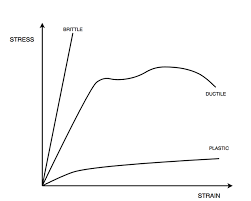The image above represents young’s modulus.

To compute for young’s modulus, two essential parameters are needed and these parameters are Stress and Strain.

The formula for calculating young’s modulus:

Young’s Modulus = Stress / Strain

Let’s solve an example;
Given that the stress is 12 and the strain is 6. Find the Young’s modulus?

This implies that;

Stress = 12
Strain = 6

Young’s Modulus = Stress / Strain
Young’s Modulus = 12 / 6
Young’s Modulus = 2

Therefore, the young’s modulus is 2.

Calculating the Stress when the Young’s Modulus and the Strain is Given.

stress = young’s modulus x strain

Let’s solve an example;
Find the stress when the young’s modulus is 32 and the strain is 10.

This implies that;

Young’s Modulus = 32
Strain = 10

stress = young’s modulus x strain
stress = 32 x 10
stress = 320

Therefore, the stress is 320.